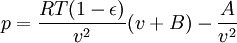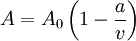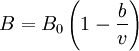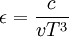Beattie-Bridgeman equation of state

The Beattie-Bridgeman equation of state is given by (Ref. 1 Eq. 1)$p= \frac{RT (1- \epsilon)}{v^2}(v+B) - \frac{A}{v^2}$

where$A = A_0 \left(1 - \frac{a}{v} \right)$$B = B_0 \left(1 - \frac{b}{v} \right)$

and$\epsilon = \frac{c}{vT^3}$

where$p$ is the pressure,$T$ is the temperature and$R$ is the molar gas constant.